# Reasoning Ability Quiz: 6 March 2021

Updated Sat, 06 Mar 2021 02:57 PM IST

Reasoning Ability Quiz For RBI Office Attendant  2021- 6th March

Q:1-5) In the following question below some statements are given followed by some conclusions. Taking the given statements to be true even if they seem to be at variance from commonly known facts, read the entire conclusion and then decide which of the given conclusion logically follows in the given statement.

Q-1)
Statement:
I) All register are book
II) All register are copy

Conclusion:
I)Some book are copy
II)All copy are book

A) Only conclusion I follows
B) Only conclusion II follows
C) Either conclusion I or II follows
D) Neither Conclusion I nor II follows
E) Both Conclusion  I and II follows

Q-2)
Statement –
I)All paper are Pen
II) No Pencil is Pen

Conclusion
I)No paper is pencil
II)Some paper is Pencil

A) Only conclusion I follows
B) Only conclusion II follows
C) Either conclusion I or II follows
D) Neither Conclusion I nor II follows
E) Both Conclusion  I and II follows

Q-3)
Statement –
I)Only a few Bike are Car
II)Some car are Truck

Conclusion
I)Some Truck are Bike
II)Some Bike are not Truck

A) Only conclusion I follows
B) Only conclusion II follows
C) Either conclusion I or II follows
D) Neither Conclusion I nor II follows
E) Both Conclusion  I and II follows

Q-4)
Statement –
I)All Red are Green
II)No Blue is Red

Conclusion:
I)some  Green is not Blue
II)Some Red are Green

A) Only conclusion I follows
B) Only conclusion II follows
C) Either conclusion I or II follows
D) Neither Conclusion I nor II follows
E) Both Conclusion  I and II follows

Q-5)
Statement:
I)Some Dog are Cat
II)Some Cat are Rat

Conclusion:
I)Some Rat are Dog
II) Some Cat are not Dog is a possibility

A) Only conclusion I follows
B) Only conclusion II follows
C) Either conclusion I or II follows
D) Neither Conclusion I nor II follows
E) Both Conclusion  I and II follows

Q: 6-10)
Direction:  Study the following arrangement carefully and answer the questions given below.
Statement:-
E  L  6  K  &  I  H  6  \$  R  S  #  M  3  E  C  @  U  4  K  ^  5  9  R  4  E  7  S  5  T

Q-6) How many such vowels are there in the given arrangement, each of which is immediately preceded by a number and immediately followed by a constant?

A)None
B)ONE
C)TWO
D)THREE
E)FOUR

Q-7) How many such numbers are there in the given arrangement, each of which is both immediately preceded and immediately followed by a consonant?

A)None
B)ONE
C)TWO
D)THREE
E)FOUR

Q-8) How many such symbol are there in the given arrangement, each of which immediately preceded by number and immediately followed by consonant?

A)None
B)ONE
C)TWO
D)THREE
E)FOUR

Q-9) Which of the following is the fourth element to the left of the twentieth element from the right end of the given arrangement?

A)E
B)H
C)@
D)I
E)None of these

Q-10) Which of the following is exactly in the middle the tenth element from the right end and the ninth element from the left end in the given arrangement?

A)E
B)C
C)3
D)M
E)U

Solutions:
Ans 1) -  A) Only conclusion I follows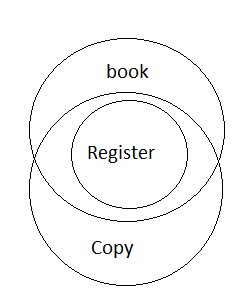Conclusion:
1. Some book are copy – follow
2. All copy are book  - Can’t say – No definite relation between book and copy

ANS-2)  Only conclusion I follows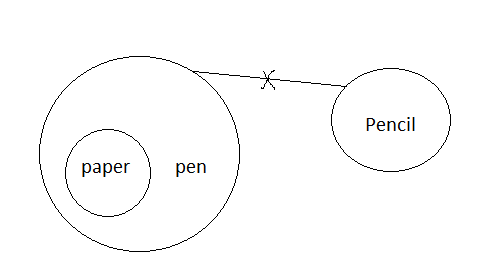Conclusion
I)No paper is pencil – Follow (as no pencil is pen )
II)Some paper is Pencil  - Does not follow (Acr. To statement it can’t be follow)

ANS-3) Either conclusion I or II follows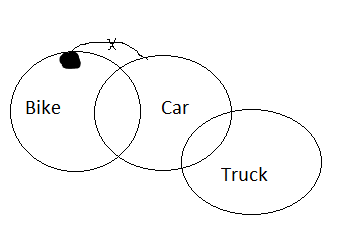Conclusion
I)Some Truck are Bike – Can’t say (No direct information)
II)Some Bike are not Truck  - Can’t say (No direct information)

ANS-4) Both Conclusion  I and II follows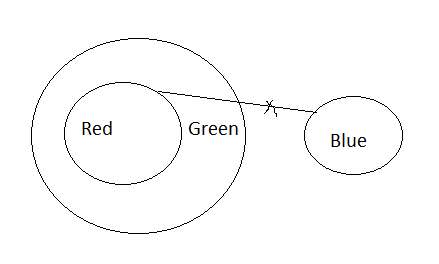Conclusion:
I)some  Green is not Blue -  Follow
II)Some Red are Green – Follow (All Red are Green )

ANS-5) Only conclusion II follows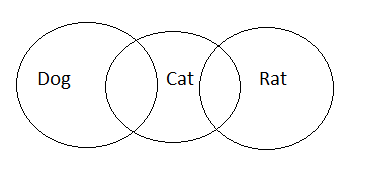Conclusion:
I)Some Rat are Dog – Can’t say (No definite relation between Dog and Rat)
II) Some Cat are not Dog is a possibility  - Follow (Some Dog are Cat hence possibility can be follow)

ANS-6)  B) ONE
Solution:
Statement:-
E  L  6  K  &  I  H  6  \$  R  S  #  M  3  E  C  @  U  4  K  ^  5  9  R  4  E  7  S  5  T
Arrangement – Number à Vowel à Consonant
Pairs - 3  E  C
Hence One is the ans

ANS-7) C)TWO
Solution:
Statement:-
E  L  6  K  &  I  H  6  \$  R  S  #  M  3  E  C  @  U  4  K  ^  5  9  R  4  E  7  S  5  T
Arrangement- consonant à Number à consonant
Pairs - L  6  K   ; S  5  T

ANS- 8)  B) one

Solution:
Statement:-
E  L  6  K  &  I  H  6  \$  R  S  #  M  3  E  C  @  U  4  K  ^  5  9  R  4  E  7  S  5  T
Arrangement- Number à symbol à consonant
Pairs : 6  \$  R

ANS-9)  B)H
Solution
Statement:-
E  L  6  K  &  I  H  6  \$  R  S  #  M  3  E  C  @  U  4  K  ^  5  9  R  4  E  7  S  5  T
20th element from right =  s
4th element to the left of s = H

ANS-10)  A) E
Solution:
Statement:-
E  L  6  K  &  I  H  6  \$  R  S  #  M  3  E  C  @  U  4  K  ^  5  9  R  4  E  7  S  5  T
10th element from the right end = ^
9th element from the left end = \$
Hence middle term is E